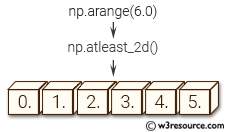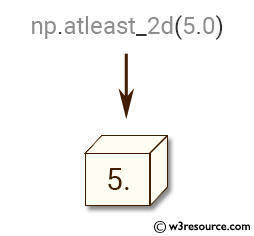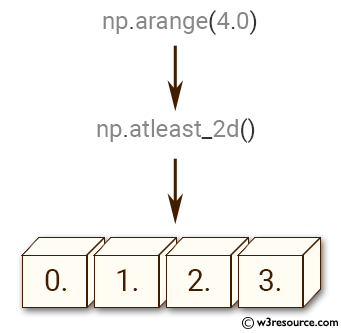# NumPy Array manipulation: atleast_2d() function

## numpy.atleast_2d() function

The numpy.atleast_2d() is used to view given inputs as arrays with at least two dimensions.

Syntax:

`numpy.atleast_2d(*arys)`Version: 1.15.0

Parameter:

Name Description Required /
Optional
arys1, arys2, . . . One or more array-like sequences. Non-array inputs are converted to arrays. Arrays that already have two or more dimensions are preserved. Required

Return value:

res, res2, . . . [ndarray]
An array, or list of arrays, each with a.ndim >= 2. Copies are avoided where possible, and views with two or more dimensions are returned.

Example-1: numpy.atleast_2d()

``````>>> import numpy as np
>>> np.atleast_2d(5.0)
array([[ 5.]])
``````

Pictorial Presentation:Example-2: numpy.atleast_2d()

``````>>> import numpy as np
>>> a = np.arange(4.0)
>>> np.atleast_2d(a)
array([[ 0.,  1.,  2.,  3.]])
``````

Pictorial Presentation:Example-3: numpy.atleast_2d()

``````>>> import numpy as np
>>> a = np.arange(4.0)
>>> np.atleast_2d(a).base is a
True
>>> np.atleast_2d(1, [1,2], [[2,3]])
[array([]), array([[1, 2]]), array([[2, 3]])]
``````

Python - NumPy Code Editor:

Previous: atleast_1d()
Next: atleast_3d()

﻿